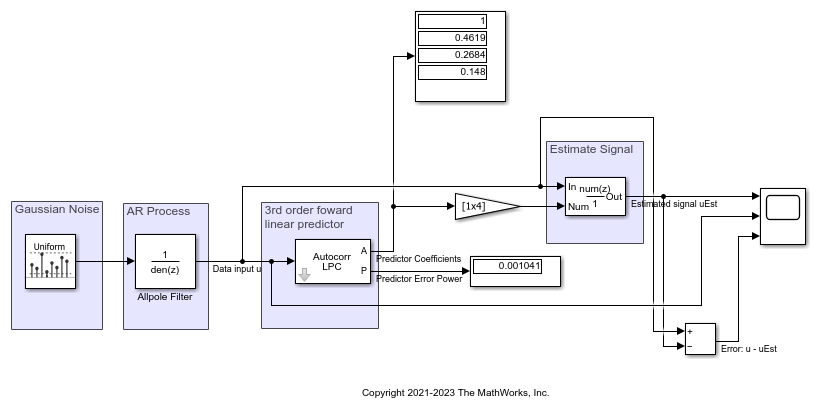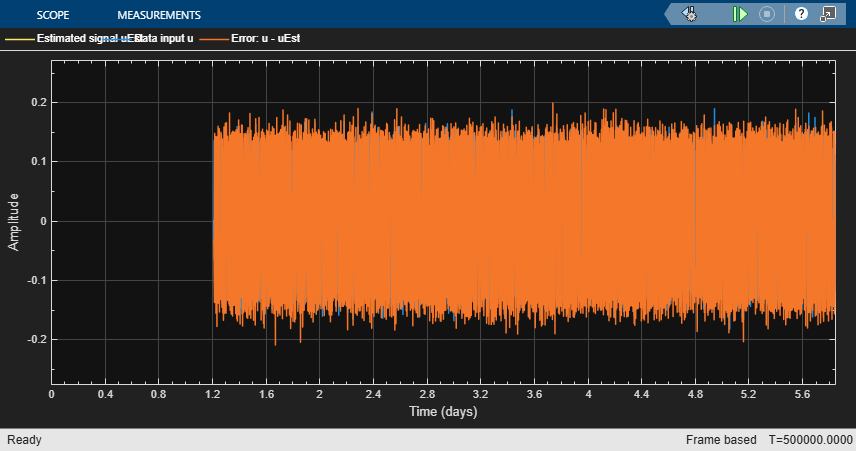# Estimate Data Series Using Forward Linear Predictor

Estimate a data series using a third-order forward linear predictor, which is modeled by the Autocorrelation LPC block. The block computes the predictor polynomial coefficients by minimizing the prediction error in the least squares sense. Estimate the future values of the signal using these predictor coefficients and past values of the signal.

First, create the signal data u as the output of a fifth order autoregressive (AR) process, driven by normalized white Gaussian noise. The noise has zero mean and a variance of 0.001.

Pass the signal data u to the Autocorrelation LPC block. The block acts as a third order forward linear predictor and outputs the coefficients of the predictor polynomial. Using these coefficients, estimate the future values of the signal u. The prediction error power is output through the Port P of the Autocorrelation LPC block. The value of this power is very close to the value of the input white noise variance indicating that the predictor polynomial coefficients are optimal. To enable the port P, you must select the Ouput prediction error power (P) in the block dialog box.

Compare the original signal and the estimated signal.